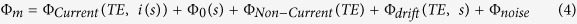## Magnetic resonance current density imaging (MRCDI) and magnetic resonance electrical impedance tomography (MREIT)

Relevance to magnetoencephalography based on magnetic resonance

Magnetic resonance current density imaging (MRCDI) and magnetic resonance electrical impedance tomography (MREIT) are two emerging techniques that combine current injection with magnetic resonance to quantify magnetic fields and determine the current density of the human brain. It could be argued that these techniques resemble magnetic resonance based magnetoencephalography.

In magnetic resonance imaging (MRI), the subject is placed in a strong static magnetic field Bz (considered to be conventionally on the z axis) which aligns the spins, resulting in a net longitudinal magnetization, represented by vector Mz.

In magnetic resonance current density imaging (MRCDI), a current applied to the head induces a magnetic field which is added (vectorially) to that of the scanner and is considered to constitute a perturbation (Göksu et al 2018). The total magnetic field B(total) can be written as a vector sum of Bz and the perturbation. The perturbation could be considered to be at an angle θ with Bz.

Such a perturbation can be analyzed in two components, one perpendicular to Bz (Bz(I)) and one parallel to it (Bz(II)). Since the perturbation is expected to be in the order of ppm, while the MRI scanner magnetic field is approximately 1.5 T, the angle θ would be approximately 0 and B(total) would be considered to be along Bz (Jog MV et al 2016 - Supplement). Similarly, the contribution of the parallel component Bz(I) is expected to be of an order than can be neglected. Therefore, the resultant magnetic field following the perturbation is considered to be along the static magnetic field of the scanner.

The above resultant magnetic field, referred to as ΔBz, will slightly change the precession frequency of the magnetization vector Mz, given that this frequency depends on the magnetic field strength and will therefore it will change the phase i.e. it will introduce a phase shift. The change of the phase will modulate the measured MRI signal proportionally to ΔBz. By measuring the phase change, we can determine the induced ΔBz and quantify the magnetic field of the brain and specifically, the magnetic field induced by the current injection. This enables the reconstruction of the inner current flow and the conductivity distribution.

This technical protocol of the study of Göksu et al 2018 uses MRI sequences i.e. sets of pulses and gradients such as multi-echo spin echo (MESE) and steady-state free precession free induction decay (SSFP-FID), with the purpose of generating an optimal signal. Representative images of SSFP-FID measurements with multi-gradient-echo readouts performed in five subjects using three different repetition times TR are shown in Figure 10 (Göksu et al 2018) where (a) shows ΔΒz(c) without current injection and (b) demonstrates ΔΒz(c) with current injection.

Figure 1: Experiment 4 (Göksu et al 2018) represents a comparison of SSFP-FID measurements with multi-gradient-echo readouts performed in five subjects using three different repetition times TR. (a) ΔΒz(c) without current injection. (b) ΔΒz(c) with current injection

The technique has been used for quantification of magnetic field changes induced in the human brain by tDCS (Jog MV et al 2016). A similar technique, magnetic resonance electrical impedance tomography (MREIT), has been used in a tACS study (Kasinadhuni AK et al 2017).

#### Technical details

As mentioned by Jog MV et al 2016, section Methods/Theory/“MRI detects induced magnetic fields”, using MRI field mapping, field variations along Bz can be measured as phase angles according to:

Φm = (γ *ΔBz * TE) mod(2π)

where Φm is the measured phase angle between 0 and 2π radians, γ is the gyromagnetic ratio, ΔBz is the field deviation along Bz and TE is the echo time. The results show that it is possible to detect magnetic field changes as small as a nanotesla (nT) with a spatial resolution of a few millimeters.

The above equation can be written as ΔBz = ΔΦm / γ x ΔTE as mentioned by (Jog MV et al 2016Supplement).

The above source also mentions that the modulo-2π operation has been removed indicating that the phase angles have been unwrapped. Δ represents the fact that we are referring to the phase difference between two TE’s. In an ideal scenario without noise, the minimum (unbiased) detectable field corresponds to the smallest possible non zero phase change generated by the smallest applied current (0.5mA). Because the full phase range of 0 to 2π radians is divided into 4096 discrete levels in MRI, the smallest non-zero phase change evaluates to 2π/4096 radians. For a given ΔTE of 9.84 msec, the minimum (unbiased) detectable field equates to 0.58nT at 0.5mA, or ~ 1.2nT/mA. It is possible to increase the ΔTE by minimizing TE1, or, increasing TE2 permitted by SNR.

It is noted that in General Linear Model Analysis the measured phase was modeled as:References

1. Göksu C, Hanson LG, Siebner HR, Ehses P, Scheffler K, Thielscher A. Human in-vivo brain magnetic resonance current density imaging (MRCDI). Neuroimage. 2018;171:26-39. https://doi.org/10.1016/j.neuroimage.2017.12.075

2. Jog MV, Smith RX, Jann K, et al. In-vivo Imaging of Magnetic Fields Induced by Transcranial Direct Current Stimulation (tDCS) in Human Brain using MRI. Sci Rep. 2016;6:34385. https://doi.org/10.1038/srep34385

3. Kasinadhuni AK, Indahlastari A, Chauhan M, Schär M, Mareci TH, Sadleir RJ. Imaging of current flow in the human head during transcranial electrical therapy. Brain Stimul. 2017;10(4):764-772. (ref.)

Additional Notes - MRI Phase and Frequency Encoding

MRI Phase encoding: The first video of link http://bit.ly/2pOaB7Z shows how an applied magnetic field gradient applied previously has changed the phase of the spins (the frequency of the circular motion is the same but the phase is different - the spins are not synchronized, "they are out of phase"). Another reference (animation):

https://www.cis.rit.edu/htbooks/mri/chap-7/g2-3.htm

MRI Frequency encoding: The first video of link http://bit.ly/31P2VPU shows how an applied magnetic field gradient changes the precession (Larmor) frequency of the spins (for the time it is applied). Another reference (animation): https://www.cis.rit.edu/htbooks/mri/chap-7/g2-2.htm